Interface Electronics

Video Lecture: 13 Oversampling Sigma Delta ADC 9.12.2020

 Video is not visible, most likely your browser does not support HTML5 video Länge: 0:0:0 Oversampling ADC 0:0:47 First order sigma delta 0:2:1 Increasing signal to noise with oversampling 0:8:47 Averaging 0:12:15 Example:100Mhz Clock, 100kHz bandwidth, 10-bit sigma-delta ADC 0:16:45 OSR=fCLK/2/fbw 0:19:27 Graph Increasing number of bits with oversampling 0:24:41 Oversampling with 1000 0:27:27 Digital droop filter 0:32:4 Problems with sigma delta modulator 0:33:7 Duty cycle and overshoot 0:35:17 DC signal and noise signal in band of interest 0:39:7 Switched capacitor circuit 0:42:22 Average current comparison 0:47:17 Simulation of SC circuit 0:49:37 Benefits and drawbacks 0:51:47 2 non overlapping clocks 0:53:27 Switched capcitor integrator 0:54:35 Second order switched capacitor circuit 0:56:12 Stability and Cascaded sigma delta (2-1-MASH) 1:2:37 Details, Comparator E(z), 1:5:7 Analog, digitized analog and digital signals 1:6:47 Integrator and switched capcitor circuit 1:17:12 z-Elements in digital domain 1:18:59 2-1 MASH sigma delta circuit 1:20:47 Jupyter notebook sigma delta 1:23:57 Delay, latency

Review and Overview

Review:
• Objectives sigma delta converter
• Oversampling, filter, aliasing, speed and resolution
• History of oversampling
• Sigma Delta converter architecture
• Passive 1st order sigma delta converter:
Schematic, SPICE, signal example, RC, simulation and filter
• Active: continous time realization (CT)
• Active: switched capacitor realization (SC)
• CIFB architecture

Droop compensating FIR filter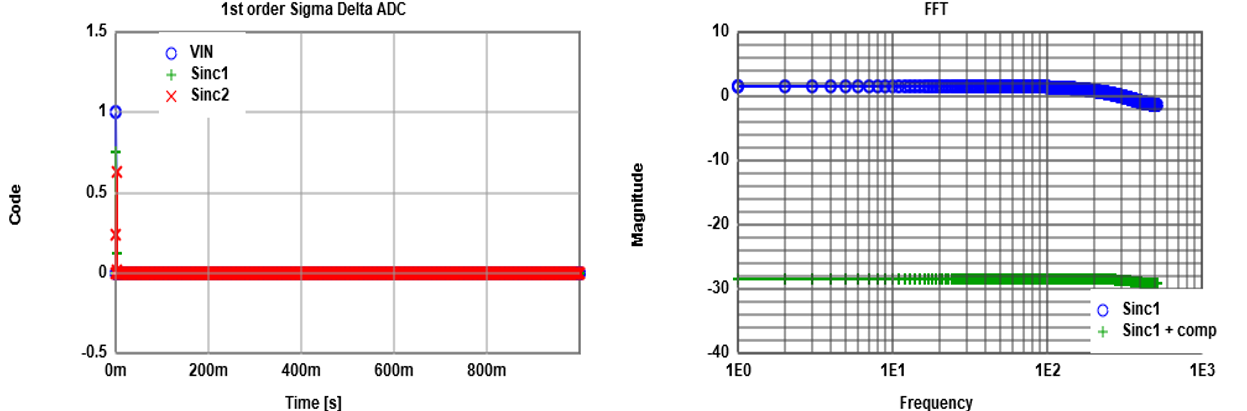The graphs are generated by 1st order passive sigma delta JavaScript simulator.
Using a pulse input the FFT shows the transfer function of the system.
Droop of the signal can be seen for higher frequencies (blue curve).
The filter can compensate for part of it (green curve).

$out(i) = \frac{-1}{K -2} in(i) + \frac{K}{K -2} in(i-1) + \frac{-1}{K -2} in(i-2)$
can be activated.
This filter function can be implemented in an FPGA employing shift, add, subtract (using 2s complement) and multiply. Fractions are normalized with the word width of the signal. K values can be taken from the link above.
 K values up to Fs/16 up to Fs/8 up to Fs/4 sinc1 25.6573 24.65659 21.04096 sinc1 error +/- dB 0.00017 0.0027 0.045 sinc2 13.79721 13.20875 11.12903 sinc2 error +/- dB 0.0004 0.0064 0.104 sinc3 9.843906 9.393567 7.835116 sinc3 error +/- dB 0.0007 0.011 0.177
Experiments:
Show transfer function: Number of periods = 0, SINC1, SINC2, + comp FIR

Input parameters:
Sample frequency: fclk
Bandwidth: fbw
Number of Bits: B

The ratio $\frac{f_{clk}}{2 \cdot f_{bw}} = OSR = K$ is called oversampling ratio.
The sigma delta converter can have an order of 1 or higher.
The electric circuit has one RC time constant per order.
The ADC needs a filter and a decimator to generate the final output stream.

Depending on the input parameters, the order (RC) and filter has to be found.
High order sigma delta modulators can give low OSR or high resolution needing more complicated filters.

• Can a 10-bit 100kHz sigma delta ADC realized using a 100 MHz clock?
• How much resolution is gained by averaging 1024 values?

• Tones in first order sigma delta modulator
• Offset is directly transfered to the output
• Mismatch causing gain error and non linearity
• Feedback DAC:
Pulsewidth, offset, Shape
The graphs can be generated by 1st order passive sigma delta JavaScript simulator.
Tone: negative value -0.651 for period generates a DC level.
Ramp test change 50% duty cycle (55%) or 0% overshoot (5%).

Switched capacitor circuits

 Source: Baker, Mixed signal design, Fig2.35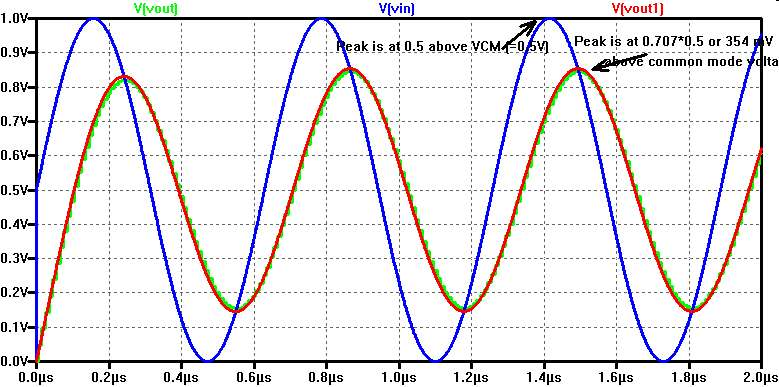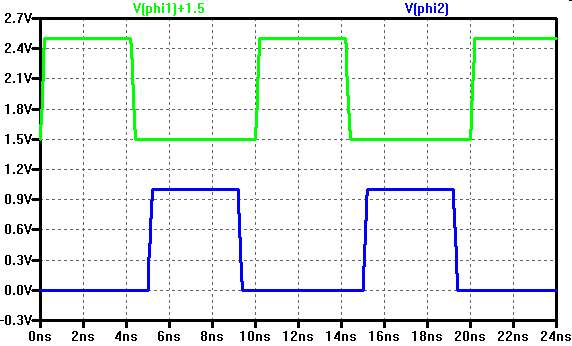In switched capacitor circuits resistors can be replaced by capacitances.
The frequency of the non overlapping clock determines the value.
Capacitances can be fabricated with high accuracy and are less temperature dependent than resistors.
The simulation is done with ideal switches.

Comparison to resistor:
What is the average current?
dV = Vin - Vout
CI · dV = Q = Iavg · dt
$I_{avg} = \frac{C_{I} \cdot dV}{dt} = C_{I} f dV = \frac{1}{R} dV = G dV$
CI f = 1p · 100MHz = 0.1 mS = G

z-domain:
$a = e^{-\frac{1}{ R C_F f}} = e^{-\frac{C_I}{C_F}}$
$H_{RC}(z) = \frac{Y(z)}{X(z)} = \frac{1-a}{1-az^{-1}}$

Reference: Take the RC Low-Pass Filter to the Z-Domain

Switched capacitor Integrator

Capacitance C1 has charge Q1.
Q1(n) = C1 * Vin
Q2(n) = Q2(n-1) + Q1(n-1)
C2 Vout(n) = C2 Vout(n-1) + C1 Vin(n-1)
C2 Vout(z) = C2 z-1 Vout(z) + C1 z-1 Vin(z)
C2 Vout(z)(1- z-1) = C1 z-1 Vin(z)
$Vout(z) = \frac{C1}{C2} \frac{z^{-1}}{1-z^{-1}} Vin(z)$
Vout(z) = H(z) · Vin(z)
$H(z) = \frac{C1}{C2} \frac{z^{-1}}{1-z^{-1}} = \frac{C1}{C2} \frac{1}{z-1}$

Second order active switched capacitor Sigma Delta modulator

 Source: Baker, Mixed signal design, Fig 7.29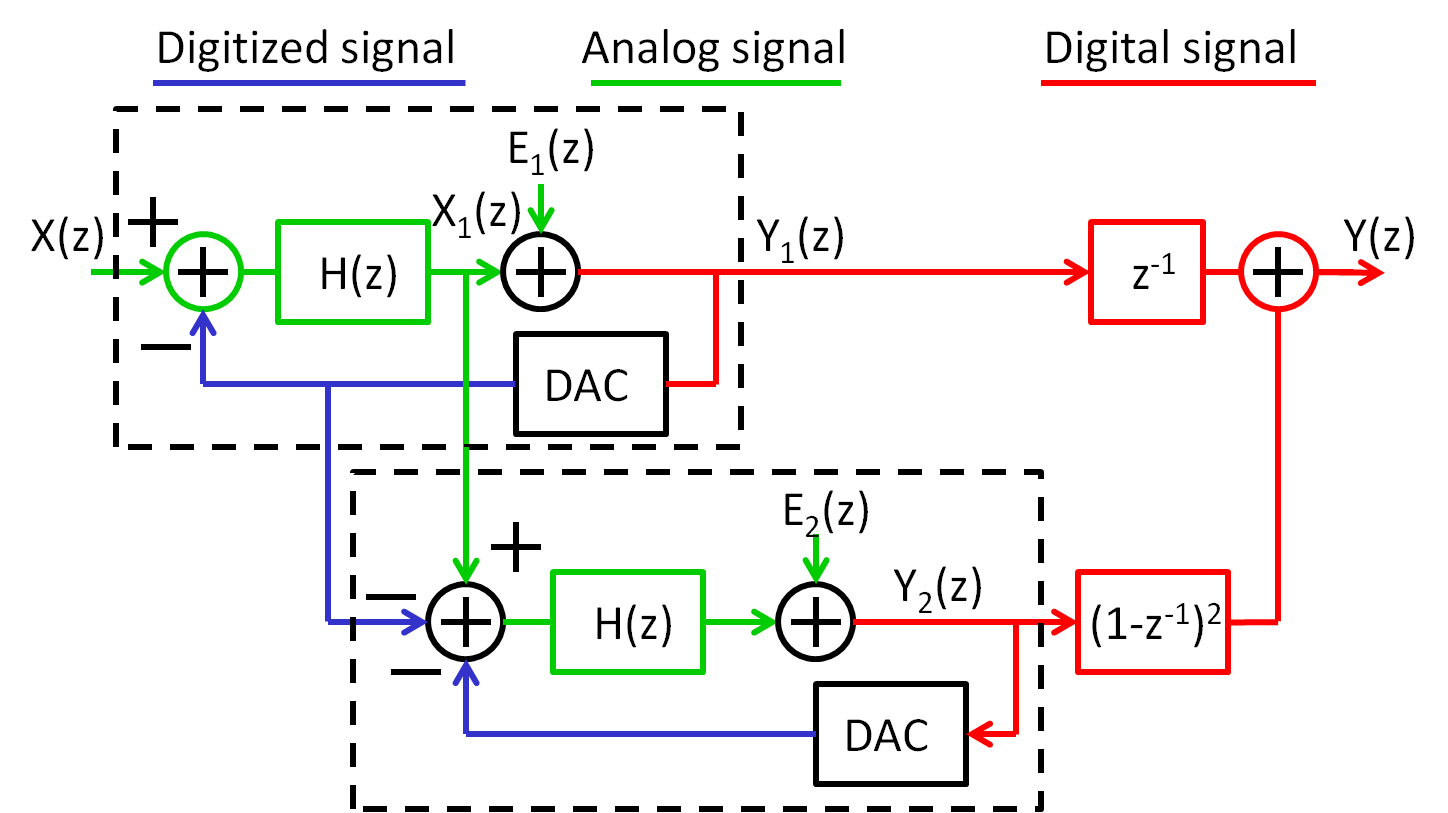Idea

Quantify digitalization error further and correct in digital domain.

The signal and noise transfer function for a 2-1 MASH sigma delta modulator can be calculated:
$Y_{1}(z) = z^{-2}\cdot X(z) + (1-z^{-1})^2\cdot E_{1}(z) \Rightarrow *z^{-1}$
$Y_{2}(z) = z^{-1}\cdot ( E_{1}(z)) + (1-z^{-1}) \cdot E_{2}(z) \Rightarrow *(1-z^{-1})^2$

$Y(z) = z^{-1}\cdot Y_{1}(z) - (1-z^{-1})^2\cdot Y_{2}(z)$
$Y(z) = z^{-3}\cdot X(z) + z^{-1} \cdot (1-z^{-1})^2\cdot E_{1}(z) - z^{-1}\cdot (1-z^{-1})^2\cdot E_{1}(z) + (1-z^{-1})^{3} \cdot E_{2}(z)$
The $E_{1}(z)$ terms cancel out:
$Y(z) = z^{-3}\cdot X(z) - (1-z^{-1})^{3} \cdot E_{2}(z)$

Brandt, Wooley, A 50-MHz Multibit Sigma Delta Modulator for 12b 2-MHz A/D Conversion, JSSC, p.1746-1756, Dec 1991

Digital z-Elements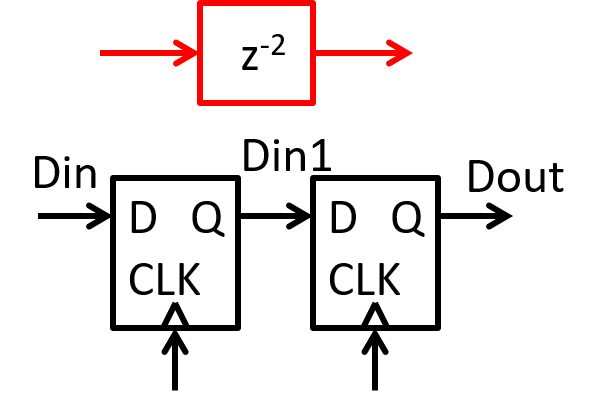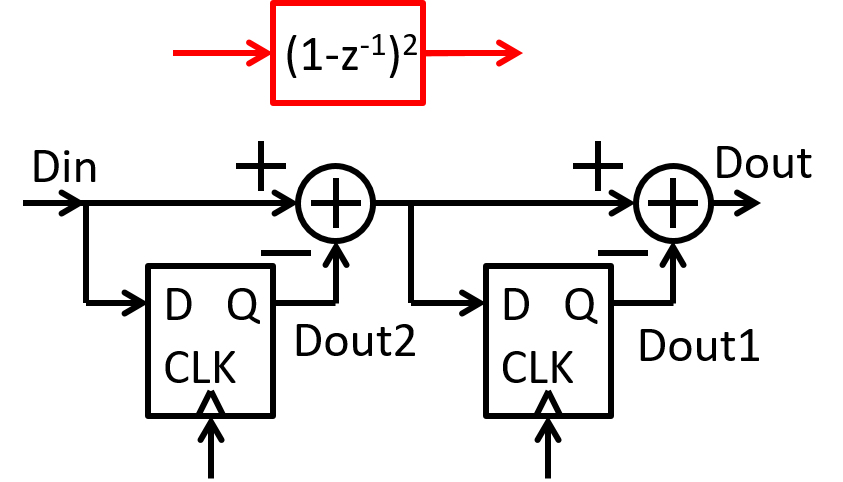JavaScript, C: Dout = Dout1; Dout1 = Din; JavaScript, C: Dout = In - Dout1 - Dout2; Dout1 = In - Dout2; Dout2 = In; VHDL: process (CLK) if risingedge(CLK) then Dout <= Dout1; Dout1 <= Din; endif; end; VHDL: Dout <= In - Dout1 - Dout2; process (CLK) if risingedge(CLK) then Dout1 <= In - Dout2; Dout2 <= In; endif; end;

A 2-1 Mash Sigma Delta ADC Circuit

Source: MSD, Baker Fig 7.58

References

"Circuit implementation of the transfer function", Akio Kitagawa, Kanazawa University
 Reference  History no Schematic 1. order passive no Simulation Full system no Digital Filter z-Plane no Digital Filter Schematic no Digital Filter VHDL no Practical Circuit no Measurement Results no Performance Data B, fC, fCLK, P

5b, 12GS/s, 81 mW ADC, El Chammas, Murrmann, VLSI 2010

"Continous Time Modulator", Mitteregger, ISSCC 2006,

"Multi-Mode Modulator", Ouzonouv, ISSCC 2007,

"Boser-Wooley Modulator", Boser & Wooley, JSSC 12/1988,

R. Gray, “Spectral analysis of sigma-delta quantization noise”

Understanding Delta-Sigma Data Converters" by Schreier and Temes (ISBN 0-471-46585-2).
CIFB Architecture, Schreier p.115

Understanding Sigma Delta Data converter, Richard Schreier

Python sigma delta resources

http://www.python-deltasigma.io/

Mapping circuit (time domain) to frequency domain representation, CMOS: Mixed-Signal Circuit Design, Second Edition, Baker, Wiley 2009
Analog Devices: Sigma Delta simulator

A first order sigma delta design example with SNR simulation

Reference: CMOS: Mixed-Signal Circuit Design

• Chapter 6: Data Converter Design Basics
• Figure 6.6/6.7: Passive RC, with RC
• Decimation Figure 6.10
• Linearity Figure 6.16
• Chapter 7: Sigma Delta
• Tones: Chapter 7.1.4
• Power supply range 7.1.5
• Limited gain: stability
• First order practical 7.1.11, Fig 7.22
• Second order practical 7.2, Fig 7.40
• MASH Modulator 7.3.6, Fig 7.58

Reference: CMOS Analog Circuit Design, Allen, Holberg

Chapter 10.9: Oversampling Converters

•  Single loop 7th order 118 dB (19 dB)
•  Single loop 5th order 20 bit:
Thomsen, Bemades, "A digitally Corrrected 20-bit Delta Sigma Modulator", ISSCC, 194-195, Feb 1994
• [60-64] 3rd order: 2-1 MASH
Longo, Copeland, "A 13-bit ISDN-Band Oversampled ADC..", CICC, pp.21.2.1-21.2.4, Jan 1988
Williams, Wooley, "A Third order Sigma-Delta..", JSSC, Vol 29. No. 3, pp.193-202,Mar 1994
Yin, Stubbe, Sansen, "Av16-bit 320 kHz CMOS ADC..", JSSC, Vol. 28, No.6, pp.640-647, June 1993
Rabii, Wooley, " A 1.8-V 0.8um CMOS ADC", JSSC, Vol 32., No. 6, pp.783-796, June 1993
Brandt, Wooley, "50MHz 12b 2MHz ADC", JSSC,Vol. 26, No. 6, pp. 1746-1756, Dec 1991
• [65-67] 4th order: 2-2 MASH
Tenhunen, "An oversampled ..", ISCAS, pp. 3279-3282, May 1990
Ritoniemi et. al., "A Stereo Audio..", JSSC, Vol. 29, No. 12, pp. 1514-1523, Dec 1994
Fujimori et al., "A 5-V 111dB dynamic range", JSSC, Vol. 32, No. 3, pp.329-336, Mar 1997
•  6th order: 2-2-2 MASH
Dedic, " A sixth order", ISSCC Dig. Tech. Papers, pp. 188-189, Feb 1994
•  MASH Decimator: Candy,Temes, oversampling Delta-Sigma Data Converters. IEEE Press
• INF4420 Projects in analog/mixed signal CMOS design